Worksheets and No Prep Teaching Resources
Critical Thinking Puzzles
Make Puzzles

# Drawing Shapes Challenge Puzzles Puzzle Workbooks for Kids - Updated Each Month!

This page contains a drawing shapes challenge, which is all about improving spatial skills. Spatial skills are a key component of critical thinking skills, but one that can be the hardest to teach. Rest assured, students' spatial skills will get a workout with these puzzles! Students are given a dot grid and directions to draw shapes within that grid. The directions are specific as to which shapes to draw, how many to draw, and placement of vertices. Several different options are available.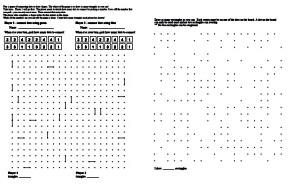Drawing Shapes Challenge

Drawing Shapes Challenge - Dot to Dot Game: Two-Player Activity Game
Students take turn drawing lines to make shapes

Drawing One Shape - Rectangles, Squares, and Triangles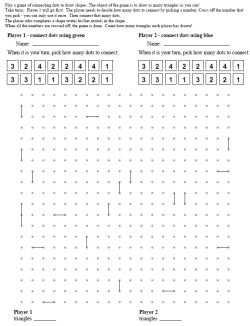Easiest: Drawing One Shape Two Player Game (Rectangles, Squares, and Triangles) - Some Lines Given Easiest: Drawing One Shape Two Player Game (Rectangles, Squares, and Triangles) - No Lines Given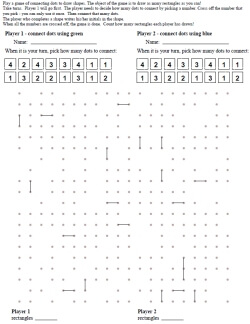Drawing One Shape Two Player Game (Rectangles, Squares, and Triangles) - Some Lines Given - With Missing Dots (25% missing) Drawing One Shape Two Player Game (Rectangles, Squares, and Triangles) - Some Lines Given - With Missing Dots (50% missing)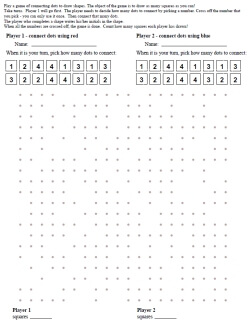Drawing One Shape Two Player Game (Rectangles, Squares, and Triangles) - No Lines Given - With Missing Dots (25% missing) Drawing One Shape Two Player Game (Rectangles, Squares, and Triangles) - No Lines Given - With Missing Dots (50% missing)

Drawing One Shape - Any ShapeEasiest: Drawing One Shape Two Player Game (Any Shape) - Some Lines Given Easiest: Drawing One Shape Two Player Game (Any Shape) - No Lines GivenDrawing One Shape Two Player Game (Any Shape) - Some Lines Given - With Missing Dots (25% missing) Drawing One Shape Two Player Game (Any Shape) - Some Lines Given - With Missing Dots (50% missing)Drawing One Shape Two Player Game (Any Shape) - No Lines Given - With Missing Dots (25% missing) Drawing One Shape Two Player Game (Any Shape) - No Lines Given - With Missing Dots (50% missing)

Drawing Two Shapes - Any Shape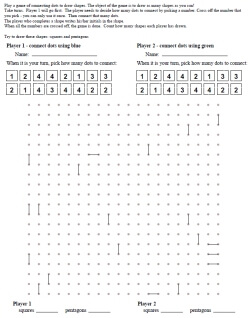Drawing Two Shapes Two Player Game (Any Shape) - Some Lines Given Drawing Two Shapes Two Player Game (Any Shape) - No Lines Given Drawing Two Shapes Two Player Game (Any Shape) - Some Lines Given - Points Given to Shapes Drawing Two Shapes Two Player Game (Any Shape) - No Lines Given - Points Given to Shapes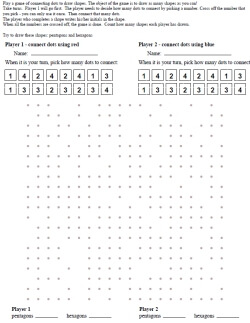Drawing Two Shapes Two Player Game (Any Shape) - No Lines Given - With Missing Dots (25% missing) Drawing Two Shapes Two Player Game (Any Shape) - No Lines Given - With Missing Dots (50% missing) Drawing Two Shapes Two Player Game (Any Shape) - No Lines Given - With Missing Dots (25% missing) - Points Given to Shapes Drawing Two Shapes Two Player Game (Any Shape) - No Lines Given - With Missing Dots (50% missing) - Points Given to Shapes

Drawing Four Shapes - Any Shape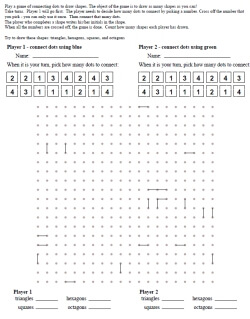Drawing Four Shapes Two Player Game (Any Shape) - Some Lines Given Drawing Four Shapes Two Player Game (Any Shape) - No Lines Given Drawing Four Shapes Two Player Game (Any Shape) - Some Lines Given - Points Given to Shapes Drawing Four Shapes Two Player Game (Any Shape) - No Lines Given - Points Given to Shapes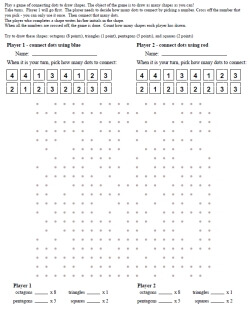Drawing Four Shapes Two Player Game (Any Shape) - No Lines Given - With Missing Dots (25% missing) Drawing Four Shapes Two Player Game (Any Shape) - No Lines Given - With Missing Dots (50% missing) Drawing Four Shapes Two Player Game (Any Shape) - No Lines Given - With Missing Dots (25% missing) - Points Given to Shapes Drawing Four Shapes Two Player Game (Any Shape) - No Lines Given - With Missing Dots (50% missing) - Points Given to Shapes

Drawing Shapes Challenge: Given One Shape and Size Requirements - Draw as many as you can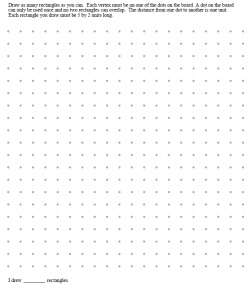Draw as many rectangles as you can - size of rectangles given Draw as many squares as you can - size of squares given Draw as many triangles as you can - size of triangles given Draw as many as you can - a shape is given (trapezoid, parallelogram, pentagon, hexagon, or octagon) Draw as many as you can - a shape is given (shape randomly picked) Four boards per page - Draw as many as you can - a shape is given (shape randomly picked)

Half of the Dots are Missing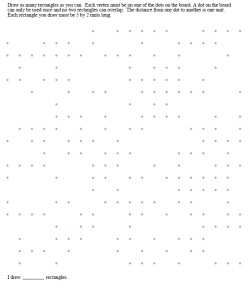Draw as many rectangles as you can - size of rectangles given Draw as many squares as you can - size of squares given Draw as many triangles as you can - size of triangles given Draw as many as you can - a shape is given (trapezoid, parallelogram, pentagon, hexagon, or octagon) Draw as many as you can - a shape is given (shape randomly picked) Four boards per page - Draw as many as you can - a shape is given (shape randomly picked)

Drawing Shapes Challenge: No Two Drawn Shapes Can be Congruent - Draw as many as you can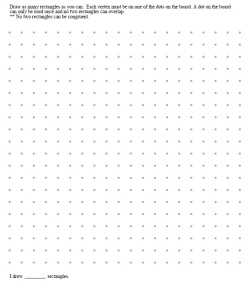Draw as many different rectangles as you can Draw as many different squares as you can Draw as many different triangles as you can Draw as many as you can - a shape is given - no two shapes drawn can be congruent (trapezoid, parallelogram, pentagon, hexagon, or octagon) Draw as many as you can - a shape is given - no two shapes drawn can be congruent (shape randomly picked)

Half of the Dots are Missing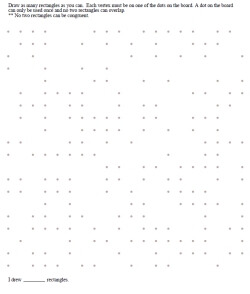Draw as many different rectangles as you can Draw as many different squares as you can Draw as many different triangles as you can Draw as many as you can - a shape is given - no two shapes drawn can be congruent (trapezoid, parallelogram, pentagon, hexagon, or octagon) Draw as many as you can - a shape is given - no two shapes drawn can be congruent (shape randomly picked)

Draw the Shapes Challenge - Shapes and How Many of the Shapes to Draw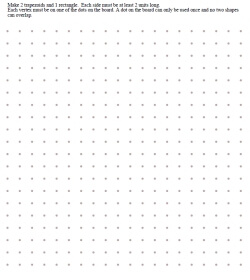Full page - drawing two different shapes Full page - drawing three different shapes Full page - drawing four different shapes Full page - drawing five different shapes Full page - drawing six different shapes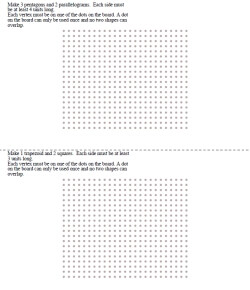Two boards per page - drawing two different shapes Two boards per page - drawing three different shapes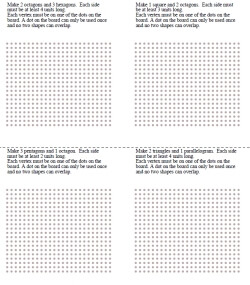Four boards per page - drawing two different shapes Four boards per page - drawing three different shapes

Area: Given One Shape and Area - Draw as many as you can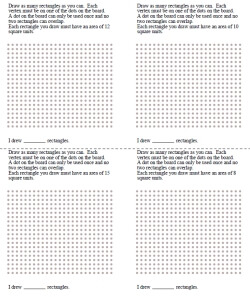Draw as many rectangles as you can (area for each rectangle is given) Draw as many squares as you can (area for each square is given)

Have a suggestion or would like to leave feedback?